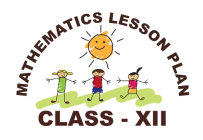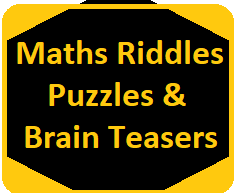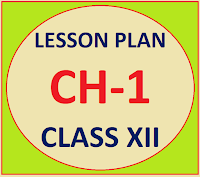## Posts

Showing posts from September, 2019

### Resource Centre Mathematics

Resource Centre Mathematics Mathematics worksheet, mathematics basic points and formulas, mathematics lesson plan, mathematics multiple choice questions Workplace Dashboard CBSE Syllabus For Session 2023-24 For  :   Classes IX & X    |   Classes XI & XII Watch Videos on Maths Solutions CLASS IX MATHEMATICS FORMULAS &  BASIC CONCEPTS

### Basic Proportionality Theorem (BPT), Thales TheoremBasic Proportionality Theorem Class 10th  (OR)  B.P.T.   or Thales Theorem   Converse of basic proportionality theorem, thales theorem 10th standard, theorem 6.2 class 10 Statement:-    If a line is drawn parallel to one side of the triangle to intersect the other two sides in two distinct points, the other two sides are divided in the same ratio. Given:-   A  Δ ABC in which line  l  ॥  BC, intersect side AB and AC at point D and E To Prove :-    Construction :-   Draw  EM 丄 AB and DN 丄 AC. Also join BE and CD Proof :-   Area of triangle =   ✕ Base ✕ Height Area of △ ADE =   ✕ AD ✕ EM ..............(1) Area of △ BDE =   ✕ BD ✕ EM ..............(2) Divide equation (1) by equation (2) we get Similarly ΔBED and ΔCDE are two triangles on the same base and lie between the same parallel DE and BC   ∴ Ar(ΔBED) = Ar(ΔCDE)   .................. (5) From equation  (3), (4), (5) we get Hence prove the Basic Proportionality Th

### Lesson Plan Math Class 12 (Ch-3) | MatricesE- LESSON PLAN    SUBJECT MATHEMATICS     CLASS 10+2 Lesson Plan Class 12th chapter 3 Matrices Subject Mathematics for Mathematics Teacher. Effective way of Teaching Mathematics. Top planning by the teacher for effective teaching in the class. Board – CBSE CLASS –XII SUBJECT- MATHEMATICS CHAPTER 3  :-  Matrices TOPIC:- Chapter : 3 : Matrices DURATION:-    This chapter is divided into eight modules and is completed in 15 class meetings. PRE- REQUISITE KNOWLEDGE:- Basic knowledge of algebra, and some simple functions. TEACHING AIDS:-   Green Board, Chalk,   Duster, Charts, smart board, projector, laptop etc. METHODOLOGY:-    Lecture method OBJECTIVES:- Introduction and concepts of matrices with different definitions, addition, subtraction, multiplication of two matrices and multiplication of matrices with a scalar. Transpose of the matrices. Symmetric and skew symmetric matrices. Concept of elemen

### Maths Riddles, Puzzles, Brain TeasersMaths Riddles, Puzzles and Brain Teasers Mathematics   Riddles,   Puzzles  and  Brain  Teasers  with answers,  Tricky  maths  riddles and number puzzles that challenges your brain power,  challenging  maths  riddles. Riddle No 1 1) If we hit a stone once then it is divided into two pieces. Then what is the number of pieces if we hit the stone for 4 times.                        Riddle No 2 2) Suppose you are given a 30 m cloth and you are asked to cut 1 m everyday from it . In how many days you completely cut the whole piece of cloth.                                                                                                   Riddle No 3 3) You are given 9 boxes and you have to write the digits from 1 to 9 in these boxes. Digits are placed in such a way so that we get a total of 15 in all directions.   Repetition  of the digit is not allowed.  Riddle No 4 4) You are given 9 boxes and you have to write the digits from 8 to 16 in th

### Lesson Plan Math Class 12 (Ch-1) Relation & FunctionsE- LESSON PLAN   SUBJECT MATHEMATICS    CLASS 10+2 Lesson Plan Class 12th Subject Mathematics for Mathematics Teacher. Effective way of Teaching Mathematics. Top planning by the teacher for effective teaching in the class. E-LESSON PLAN MATHEMATICS CLASS-XII CHAPTER-1 RELATIONS & FUNCTIONS NAME OF THE TEACHER DINESH KUMAR CLASS 10+2 CHAPTER 01 SUBJECT MATHEMATICS TOPIC RELATIONS & FUNCTIONS  DURATION : 15 Class Meetings PRE- REQUISITE KNOWLEDGE:- Knowledge of set theory chapter 1 class 10+1 Knowledge of Relations and Functions chapter 2 class 10+1 TEACHING AIDS:-   Green Board, Chalk,    Duster, Charts, smart board, projector, laptop etc. METHODOLOGY:-     Lecture cum demonstration and Learning by doing method LEARNING OBJECTIVES  To explain the Cartesian product and different types of relations. Reflexive, symmetric, transitive and equivalence